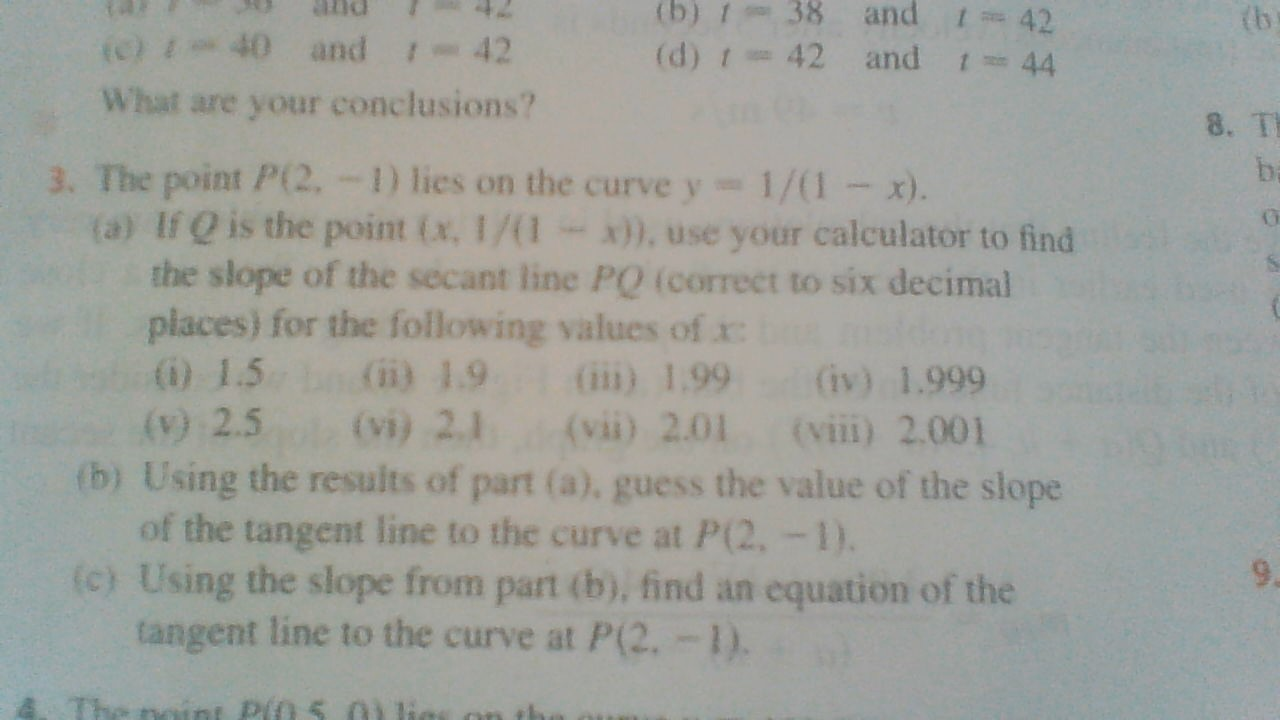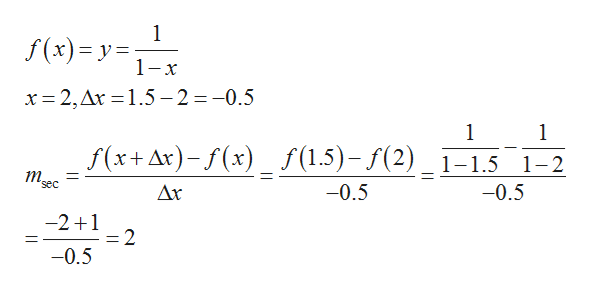and t(b) 38(d) r42 and(b42(o) 40 and -4244What are your conclusions?8. T3. The point P(2, -1) lies on the curve y(a) If Q is the point (x. 1/(1the slope of the secant line PQ (correct to six decimalplaces) for the following values of x:(0 1.51/(1 x).x)), use your calculator to findi) 1.9(vi) 2.1i) 1.99 (iv) 1.999(vii) 2.01 (viii) 2.001() 2.5(b) Using the results of part (a), guess the value of the slopeof the tangent line to the curve at P(2, -1).(c) Using the slope from part (b), find an equation of thetangent line to the curve at P(2. -1).noint Pi0 5 01 lio

Question

I am doing homework for Calculus and I am having trouble with #3 tangent problem on Secction 2.1 of the James Stewart Calculus Eighth Edition, page 82. I need some hints on what to do on the first (a) part of the question, please?help_outlineImage Transcriptioncloseand t (b) 38 (d) r42 and (b 42 (o) 40 and -42 44 What are your conclusions? 8. T 3. The point P(2, -1) lies on the curve y (a) If Q is the point (x. 1/(1 the slope of the secant line PQ (correct to six decimal places) for the following values of x: (0 1.5 1/(1 x). x)), use your calculator to find i) 1.9 (vi) 2.1 i) 1.99 (iv) 1.999 (vii) 2.01 (viii) 2.001 () 2.5 (b) Using the results of part (a), guess the value of the slope of the tangent line to the curve at P(2, -1). (c) Using the slope from part (b), find an equation of the tangent line to the curve at P(2. -1). noint Pi0 5 01 lio fullscreen
Step 1

The method to find the slope of secant ...help_outlineImage Transcriptionclose1 f(x) y 1-x x 2, Ar 1.5 -2 =-0.5 1 1 f(x+Ar)(x)_f(1.5)- f(2)_1-1.5 1-2 m. sec -0.5 -0.5 Δx -2 1 2 -0.5 fullscreen

Want to see the full answer?

See Solution

Want to see this answer and more?

Our solutions are written by experts, many with advanced degrees, and available 24/7

See Solution
Tagged in

Other